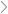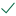Questions & AnswersStatistics and Probabilityhe weights of 5 squash (in pounds) are 10,17,17.5,18.5,...   The...

QuestionAnswered step-by-step

# he weights of 5 squash (in pounds) are 10,17,17.5,18.5,...   The...

he weights of 5 squash (in pounds) are 10,17,17.5,18.5,...

The weights of 5 squash (in pounds) are 10,17,17.5,18.5, and 19.5

. The sample weights have a mean of x¯=16.5

. The accepted population standard deviation for this type of squash is σ=1.25

a. What is the margin of error at the 90% confidence level? Type as #.##

b. What is the margin of error at the 99% confidence level? Type as: #.##

c. What is the 90% confidence interval? Type as: [##.##, ##.##]

d. What is the 99% confidence interval? Type as: [##.##, ##.##]

A tax assessor assesses the mean property tax bill for all property owners in a certain city. A recent survey obtained a sample mean of 1400 dollars. The population standard deviation is known to be 1000 dollars.

a. How many tax records should be obtained at a 90% confidence level to have a margin error of 100 dollars? Round up to the nearest whole number.

b. If the population standard deviation goes up to 1500, would the margin of error be equal, greater than, or less than 100? Type as: equal, greater than, or less than

The weights of pumpkins (in pounds) of 6 pumpkins are 5,7,7.5,8,8.5,and 8.75. The sample weights have a mean of x¯=7.458 and a standard deviation of s=1.245

a. What is the degrees of freedom?

b. What is the critical value when the significance level is α=0.1? Type as: #.###

c. What is the margin of error? Type as: #.###

d. What is the 90% t-confidence interval for pumpkin weights? Type as: [#.### , #.###]Solved by verified expert

sectetur adipiscing elit. Nam lacinia pulvinar tortor nec facilisis. Pellente

sectetur adipiscing elit. Nam lacinia pulvinar

sectetur adipiscing elit. Nam lacinia pu

sectetur adipiscing elit. Nam lacinia pulvinar tortor nec10,000 step-by-step explanations

## Step-by-step explanation

sectetur adipiscing elit. Nam lacinia pulvinar tortor nec facilisis. Pellentesque dapibus e

sectetur adipiscing elit. Nam lacinia pulvinar tortor nec facilisis. Pellentesque dapibus

sectetur adipiscing elit. Nam lacinia pulvinar tortor nec facilisis. Pellentesque d